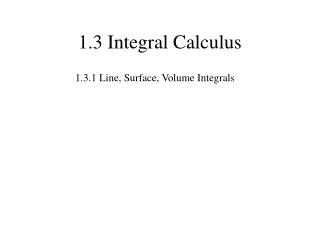Download Presentation1.3 Integral Calculus

# 1.3 Integral Calculus

Download Presentation## 1.3 Integral Calculus

- - - - - - - - - - - - - - - - - - - - - - - - - - - E N D - - - - - - - - - - - - - - - - - - - - - - - - - - -
##### Presentation Transcript

1. 1.3 Integral Calculus 1.3.1 Line, Surface, Volume Integrals

2. a) line integral:

3. Example 1.6

4. For a given boundary line there many different surfaces, on which the surface integral depends. It is independent only if If the surface is closed: b) surface integral:

5. 2 2 2 Example 1.7

6. volume integral:

7. Example 1.8

8. 1.3.3 Fundamental Theorem for Gradients The line integral does not depend on the path P.

9. Example 1.9 along I-II and III

10. 1.3.4 Fundamental Theorem for Divergences (also Gauss’s or Green’s theorem) The surface S encloses the volume V.

11. dz dy dx

12. Example 1.10 Check the divergence theorem for

13. 1.3.5 Fundamental Theorem for Curls (also Stokes’ theorem) The path P is the boundary of the surface S. The integral does not depend on S.

14. dz dy

15. You must do it in a consistent way!

16. Example 1.11 Check Stokes’ Theorem for